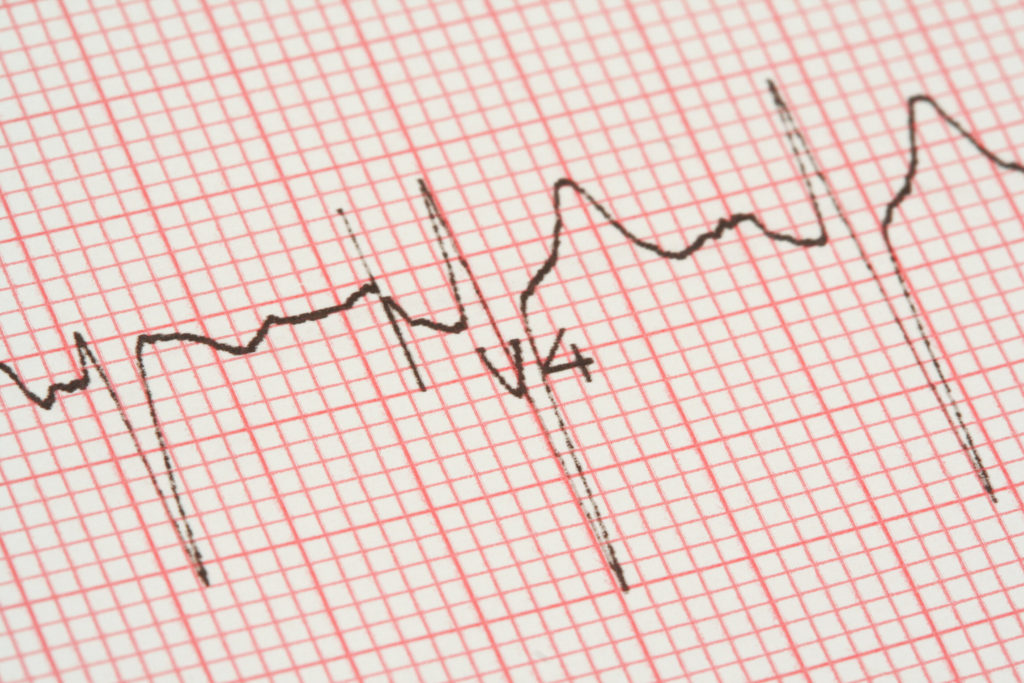# Measuring the Amplitude of an ECG Deflection

I was recently asked a question regarding the amplitude of a deflection on an ECG – more specifically, the question was about how to determine the amplitude of a deflection. When we say amplitude, we really mean magnitude.

First of all, it is very important to understand that every deflection on the ECG is a vector and also that every vector has direction and magnitude. Our measurements of a vector’s direction also occur at one instance in time. Vectors can change directions – up, down, right, left. They can even travel in circles. But at each instance in time they are traveling in one specific direction.

The ECG itself is a graph of these vectors with time represented on the horizontal axis and voltage on the vertical axis. We are trained to view voltage as the measurement of the amplitude of a vector. However, the amplitude of a deflection (again, all deflections are vectors) is actually the area under the curve using the baseline to serve as the third side thus enclosing the area. Any attempt to use the height or depth of a deflection as the amplitude of that deflection is only going to be a very rough approximation of the amplitude…  somewhat analogous to determining the volume of a box by considering only how tall the box is. What if the box is short but very, very wide? How would that compare with a box that was just slightly taller but very, very narrow?

Unless you are a genius at determining the formula for the slope of that deflection and can then determine the area under the curve using integral calculus… using the height or depth as measured on the vertical axis is just about the best we can do. In the case of a QRS interval that may contain both positive and negative deflections, you should allow for the algebraic addition of the positive and negative areas, i.e., a QRS with a large Q wave and a large S wave but with a very small r wave is going to have a “negative” amplitude. Actually, the amplitude itself is not negative, but the vertical axis also indicates direction, hence the “negative” result. If possible, you should also allow for the width of each individual deflection for greater accuracy. This gets rather complicated.

In the “old” days, Braunwald, Marriott and others would try to count the squares contained within a deflection for a better approximation of the amplitude (they even estimated partial squares!). This is obviously not feasible in today’s busy practices. The best we can do is measure the height or depth of the deflection, often considering its width as well. This is why you sometimes see the ECG machine giving a mean axis in the frontal plane that is based on a QRS that does not appear to be the tallest of all the limb leads.

Today, the amplitude of a deflection (i.e., magnitude of a vector) is used to determine the frontal plane axis of that deflection, the origin of a P wave, the acuity of a subendocardial and/or epicardial ischemia and the size of a heart chamber.

Just remember that using the height or depth of a deflection to determine amplitude (magnitude) – which is the best we can do – is like estimating the volume of a box using only its height. Always be aware of the inherent vagaries of determining amplitude by this method.This site uses Akismet to reduce spam. Learn how your comment data is processed.